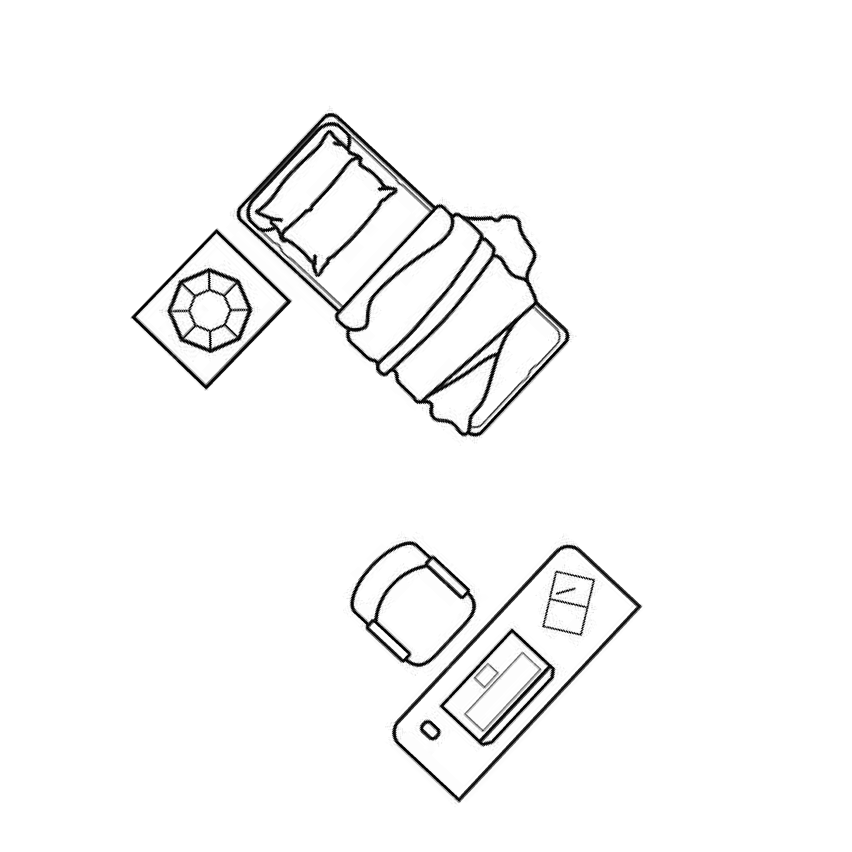IntroductionIntroduction# Perimeter of Polygons

INTRO
Perimeter is the total distance around the border of a 2D shape. To find the perimeter of a polygon, we need to find the length of each of its sides, then add them all together.
To help us visualize this process, let's imagine we want to brighten up a room by lining it with some string lights.The perimeter is like the total length of string lights we need to line the walls.
If each of our walls has length , then we need a total string light length of to line the room.So the perimeter of this room is also equal to . Perimeters are lit ✨.Check out our
Calculator
or explore our
Lesson
and
Practice

CALCULATOR

## Perimeter of Polygons Calculator

### Step 1. Determine whether the polygon is regular.

The shape is regular if all the sides are the same length.
KEY STEPS

## How to Find the Perimeter of Polygons

### Step 1. Determine whether the polygon is regular.

The shape is regular if all the sides are the same length.

### Step 3. Determine the lengths of each side of the polygon.

If the shape is regular, all the sides have the same length, so you only need to know the length of one side to calculate the perimeter.
If the shape is not regular, make sure you find the length of every side you counted in Step 2.

### Step 4. Add up the side lengths.

If the shape is regular, just multiply the length of one side by the number of sides.
If the shape is not regular, add up all the side lengths you found in Step 3.
LESSON
Perimeter of Polygons

## Perimeter of Polygons

Finding the perimeter of a polygon is like finding the total length of string lights needed to line a room.
If we have a regular polygon, meaning the sides are all the same length, we only need to find the length of one side and then multiply it by the number of sides:
PRACTICE
Perimeter of Polygons

## Practice: Perimeter of Polygons

Question 1 of 8: Find the perimeter of this regular polygon:

#### Step 1. Determine whether the polygon is regular.

The shape is regular if all the sides are the same length. What is this shape?

CONCLUSION
Incredible job, look at you go! Thanks for checking out this lesson ☺️🙏. Where to next?Leave Feedback